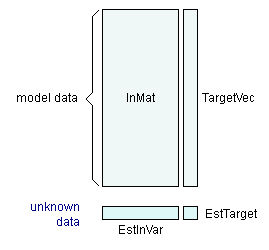The SDL Component Suite is an industry leading collection of components supporting scientific and engineering computing. Please visit the SDL Web site for more information....## EstimateByKNN

 Unit: SDL_math2 Class: none Declaration: procedure EstimateByKNN (InMat: TMatrix; TargetVec: TVector; kn: integer; WeightingMode: TKnnWMode; SmoothFact: double; EstInVar: TVector; var EstTarget: double; var EstMeanDist: double);

The procedure EstimateByKNN estimates an unknown value by using a KNN model. The KNN model data is stored in the parameters InMat and TargetVec. The parameter InMat contains the independent variables of the training data, the vector TargetVec contains the target data of the training set. The model is set up using kn nearest neighbors. In order to estimate the dependent variable EstTarget of the unknown data EstInVar, one of five different weighting modes can be selected using the parameter WeightingMode. The variable parameter EstMeanDist returns the mean distance of the kn neighbors used to calculate EstTarget.

The weighting mode as defined by the parameter WeightingMode determines the performance of the estimator. The weighting modes kwmLinear, kwmBiQuad, and kwmGauss should be used for continuous target variables (i.e. for function approximation problems), while the mode kwmMedian is to be used for discrete target variables (i.e. for classification problems). The mode kwmAverage falls somewhere in between these two extremes. The parameter SmoothFact is only used for kwmGauss and kwmLinear weighting modes, otherwise the value of SmoothFact is ignored. The lower SmoothFact is set, the smoother is the generated estimation surface. Please note that smooth estimates tend to average out (perhaps important) details of the model data.On the other hand too little smoothing leaves too much room for noise in the data, creating noisy and unreliable estimates. A good compromise is to set SmoothFact to values between 1.2 and 1.7.

The relationships among the model parameters are shown in the following diagram:Hint: The number of nearest neighbors kn must not be greater than the number of training data (i.e. InMat.NrOfRows ). If so, kn is automatically reduced to this value.

 Example: This procedure is used in the following example program (see http://www.lohninger.com/examples.html for downloading the code): interpol3d

Last Update: 2013-Mar-31